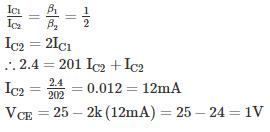Courses

# Analog Electronics - 4

## 10 Questions MCQ Test RRB JE for Electronics & Communication Engineering | Analog Electronics - 4

Description
This mock test of Analog Electronics - 4 for Electronics and Communication Engineering (ECE) helps you for every Electronics and Communication Engineering (ECE) entrance exam. This contains 10 Multiple Choice Questions for Electronics and Communication Engineering (ECE) Analog Electronics - 4 (mcq) to study with solutions a complete question bank. The solved questions answers in this Analog Electronics - 4 quiz give you a good mix of easy questions and tough questions. Electronics and Communication Engineering (ECE) students definitely take this Analog Electronics - 4 exercise for a better result in the exam. You can find other Analog Electronics - 4 extra questions, long questions & short questions for Electronics and Communication Engineering (ECE) on EduRev as well by searching above.
QUESTION: 1

### The feedback topology of the given circuit is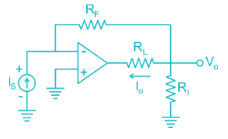Solution:

The input is current, current is added in parallel (Hence shunt).

From the output, voltage is sampled (shunt)

Hence shunt series feedback topology.

*Answer can only contain numeric values
QUESTION: 2

### In the circuit shown below all the transistors are identical with; VBE = 0.7 V; VCE(sat) = 0.2 If the transistor Q3 is in saturation then V0 is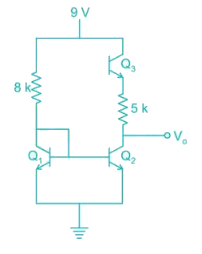Solution:

The collector currents of both Q1 and Q2 will be identical due to same base current.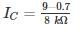Since Q3 is in saturation

9 – 0.2 – 5 × 103 IC - VO = 0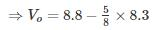Vo = 3.6 V

QUESTION: 3

### Consider series-shunt amplifier in which the open loop gain is Av = 105 and the closed loop gain is AVf = 50. Assume the input and output resistance of the basic amplifier are Ri = 10 kΩ and Ro = 20 kΩ. Determine the input resistance of series input connecting and the output resistance of a shunt output connection for an ideal feedback voltage amplifier.

Solution:

The ideal closed-loop voltage transfer function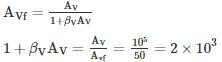The input resistance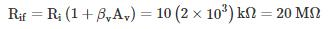The output resistance is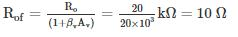QUESTION: 4

For the circuit shown in fig, the transconductance of Q1 is gm1, of Q2 is gm2 and Q3 is gm3 then the overall trans-conductance gm is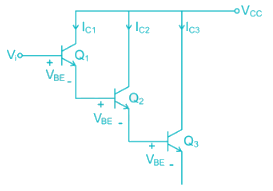Solution: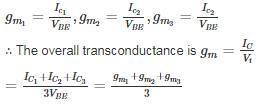*Answer can only contain numeric values
QUESTION: 5

The circuit shown in figure uses matched transistor. With a thermal voltage VT = 25 mV, The value of β is very high. Find the value of emitter resistance in kΩ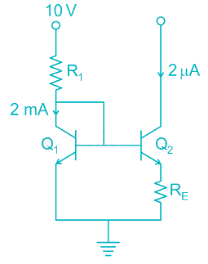Solution: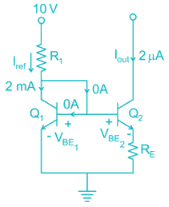Given circuit is widlar current source which is particularly suitable for low value of current.

Here Iout ≠ Iref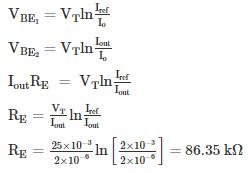QUESTION: 6

The two stages of the amplifier are shown in the figure. Both transistors have current gain β of 80 and dynamic emitter resistance r′e of 25Ω. The magnitude of the overall voltage gain of the amplifier is approximately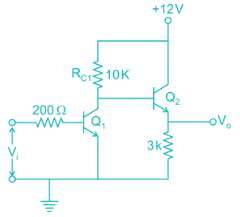Solution:

Objective approach

The second stage is emitter follower which has voltage gain of unity.

The overall voltage gain = gain of CE stage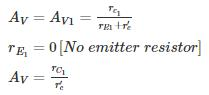rC1 = effective impedance seen by collector of transistor Q1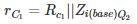Zi(base)Q2 at the base of transistor Q2.
The impedance Zi (base) Q2 at the base of transistor Q is
Zi(base)2 = βRE2
= 80 × 3 k

Zi (base) 2 = 240 kΩ

Therefore: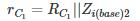= 10 k||240 k

rC1 = 9.6kΩ

Overall voltage gain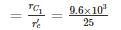AV− = 3 84

Subjective approach:

Draw the equivalent h-parameter model

rπ = β re = 80 x 25 = 2k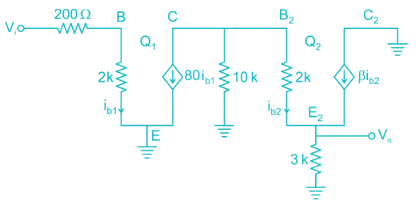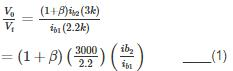Apply KVL in the base-emitter loop of transistor Q2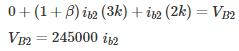Apply KCL at node VB2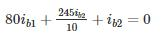800 ib1 = -255  ib2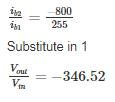*Answer can only contain numeric values
QUESTION: 7

Two RC coupled amplifiers are connected to form a 2-stage amplifier. If the lower and upper cut off frequencies of each individual amplifiers is 100 Hz and 20 kHz. Then the 3-dB bandwidth of the two-state amplifier is ____ Hz.

Solution:

Concept:

For multistage amplifier consisting of n identical amplifying states, the lower cut off fL (multi) is expressed as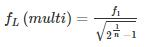Where f1 = lower cut off frequency of individual state.

Upper cut off frequency is given by: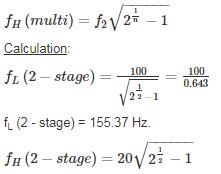= 20 × 0.643

= 12.87 kHz

Bandwidth =

= 12714.6 Hz.

QUESTION: 8

In the AC equivalent circuit shown in Fig, if Iin is the Input current and Feedback Resistance is very large, the type of Feedback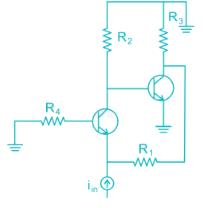Solution:

Procedure to identify the type of Feedback:

(1) Identify the element responsible for the Feedback.

(2) If Feedback element is directly connected to the output node, it indicates voltage sampling otherwise it indicates current sampling.

(3) If Feedback element is directly connected to the Input node it indicates shunt Mixing otherwise it indicate series mixing.

∴ It is voltage current Feedback.

*Answer can only contain numeric values
QUESTION: 9

The differential DC output voltage V01 – V02 in the circuit shown is ____ V.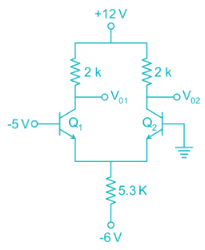Solution:

The base of Q1 is biased with negative voltage hence

Q1 → OFF

Q2 → ON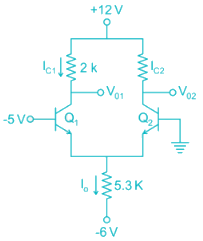IC1 = 0

V01 = 12 V

VE2 = -0.7 V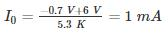V02 = 12 – (1 mA) (2k)

= 12 – 2

= 10 V

V01 – V02 = 12 – 10 = 2 V

QUESTION: 10

Find the value of VCE in the given figure.
Assume the base currents to be the same.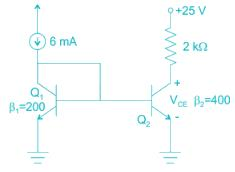Solution: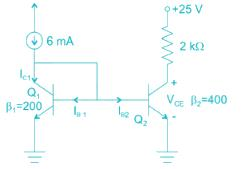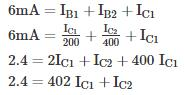Also we know IC = βIB

Since base currents are the same, we have